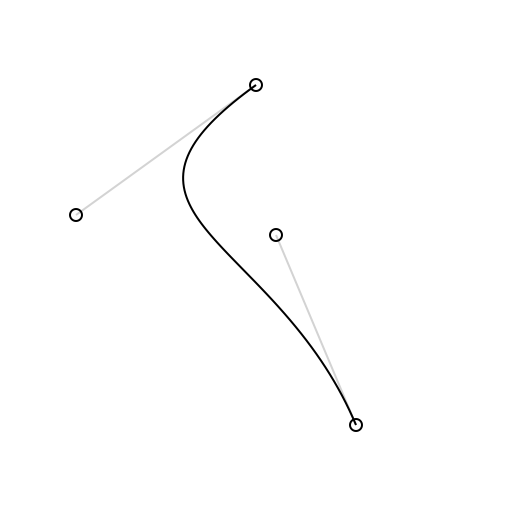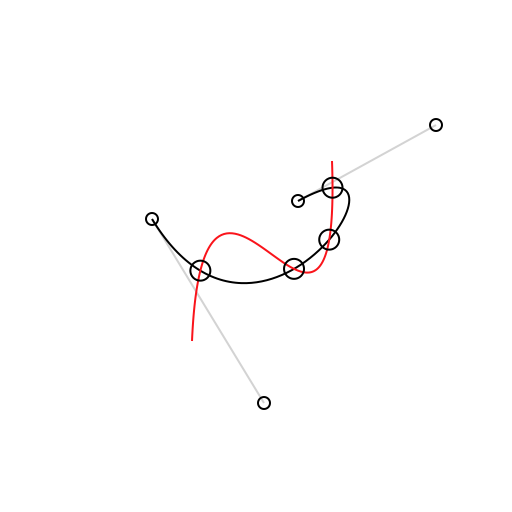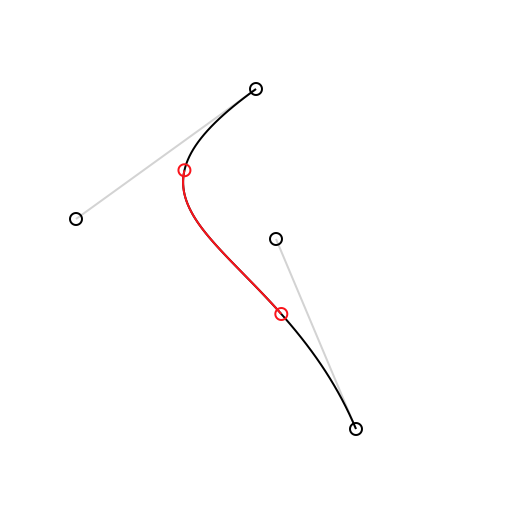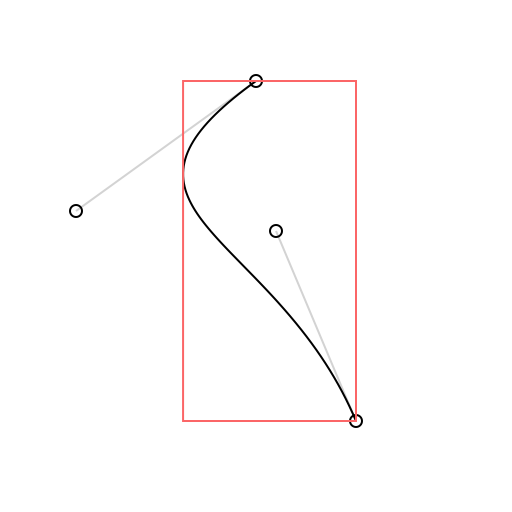Showing:

0

136

4mos ago

3

0

MIT

# BezierKit

BezierKit is a comprehensive Bezier Path library written in Swift.

## Warning! Prerelease software!

Please note that BezierKit is currently pre-release software. Its releases follow semantic versioning which means that until it reaches 1.0 status the API may not be stable or backwards compatible.

## Features

• Constructs linear (line segment), quadratic, and cubic Bézier curves
• Draws curves via CoreGraphics
• Determines positions, derivatives, and normals along curves
• Lengths of curves via Legendre-Gauss quadrature
• Intersects curves and computes cubic curve self-intersection to any degree of accuracy
• Determines bounding boxes, extrema,
• Locates nearest on-curve location to point
• to any degree of accuracy
• Splits curves into subcurves
• Offsets and outlines curves
• Comprehensive Unit and Integration Test Coverage
• Complete Documentation

## Installation

### CocoaPods

CocoaPods is a dependency manager for Cocoa projects. You can install it with the following command:

``````\$ gem install cocoapods
``````

To integrate BezierKit into your Xcode project using CocoaPods, add it to your target in your `Podfile`:

``````target '<Your Target Name>' do
pod 'BezierKit', '>= 0.11.0'
end
``````

Then, run the following command:

``````\$ pod install
``````

### Swift Package Manager

The Swift Package Manager is a tool for automating the distribution of Swift code and is integrated into the `swift` compiler. Once you have your Swift package set up, adding BezierKit as a dependency is as easy as adding it to the `dependencies` value of your `Package.swift`.

``````// swift-tools-version:5.0
import PackageDescription

let package = Package(
dependencies: [
.package(url: "https://github.com/hfutrell/BezierKit.git", from: "0.10.0"),
]
)
``````

## Usage

### Constructing & Drawing Curves

BezierKit supports cubic Bezier curves (`CubicCurve`) and quadratic Bezier curves (`QuadraticCurve`) as well as line segments (`LineSegment`) each of which adopts the `BezierCurve` protocol that encompasses most API functionality.``````import BezierKit

let curve = CubicCurve(
p0: CGPoint(x: 100, y: 25),
p1: CGPoint(x: 10, y: 90),
p2: CGPoint(x: 110, y: 100),
p3: CGPoint(x: 150, y: 195)
)

let context: CGContext = ...       // your graphics context here
Draw.drawSkeleton(context, curve)  // draws visual representation of curve control points
Draw.drawCurve(context, curve)     // draws the curve itself
``````

### Intersecting Curves

The `intersections(with curve: BezierCurve) -> [Intersection]` method determines each intersection between `self` and `curve` as an array of `Intersection` objects. Each intersection has two fields: `t1` represents the t-value for `self` at the intersection while `t2` represents the t-value for `curve` at the intersection. You can use the `ponit(at:)` method on either of the curves to calculate the coordinates of the intersection by passing in the corresponding t-value for the curve.

Cubic curves may self-intersect which can be determined by calling the `selfIntersections()` method.``````let intersections: [Intersection] = curve1.intersections(with: curve2)
let points: [CGPoint] = intersections.map { curve1.point(at: \$0.t1) }

Draw.drawCurve(context, curve: curve1)
Draw.drawCurve(context, curve: curve2)
for p in points {
Draw.drawPoint(context, origin: p)
}
``````

### Splitting Curves

The `split(from:, to:)` method produces a subcurve over a given range of t-values. The `split(at:)` method can be used to produce a left subcurve and right subcurve created by splitting across a single t-value.``````Draw.setColor(context, color: Draw.lightGrey)
Draw.drawSkeleton(context, curve: curve)
Draw.drawCurve(context, curve: curve)
let subcurve = curve.split(from: 0.25, to: 0.75) // or try (leftCurve, rightCurve) = curve.split(at:)
Draw.setColor(context, color: Draw.red)
Draw.drawCurve(context, curve: subcurve)
Draw.drawCircle(context, center: curve.point(at: 0.25), radius: 3)
Draw.drawCircle(context, center: curve.point(at: 0.75), radius: 3)
``````

### Determining Bounding Boxes``````let boundingBox = curve.boundingBox
Draw.drawSkeleton(context, curve: curve)
Draw.drawCurve(context, curve: curve)
Draw.setColor(context, color: Draw.pinkish)
Draw.drawBoundingBox(context, boundingBox: curve.boundingBox)
``````

### More

BezierKit is a powerful library with a lot of functionality. For the time being the best way to see what it offers is to build the MacDemos target and check out each of the provided demos.

## Rate & Review

Great Documentation0
Easy to Use0
Performant0
Highly Customizable0
Bleeding Edge0
Responsive Maintainers0
Poor Documentation0
Hard to Use0
Slow0
Buggy0
Abandoned0
Unwelcoming Community0
100